# HW 4 EECS 110 Problem 2 n For

• Slides: 17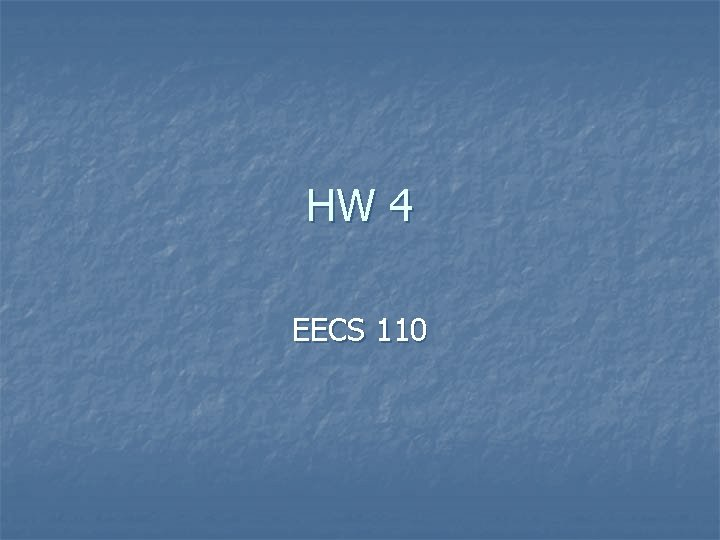HW 4 EECS 110Problem #2 n For this problem, you will implement a (text-based) menu of options for analyzing a list of stock prices (or any list of floating-point values). The top-level function to write is called tts() -- it takes no inputs. Instead, it offers the user a menu with these choices: (0) Input a new list (1) Print the current list (2) Find the average price (3) Find the standard deviation (4) Find the min and its day (5) Find the max and its day (6) Your TT investment plan (9) Quit n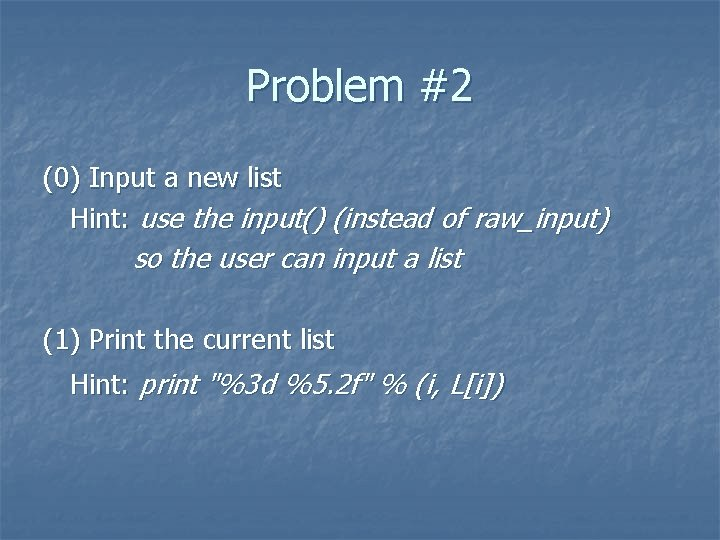Problem #2 (0) Input a new list Hint: use the input() (instead of raw_input) so the user can input a list (1) Print the current list Hint: print "%3 d %5. 2 f" % (i, L[i])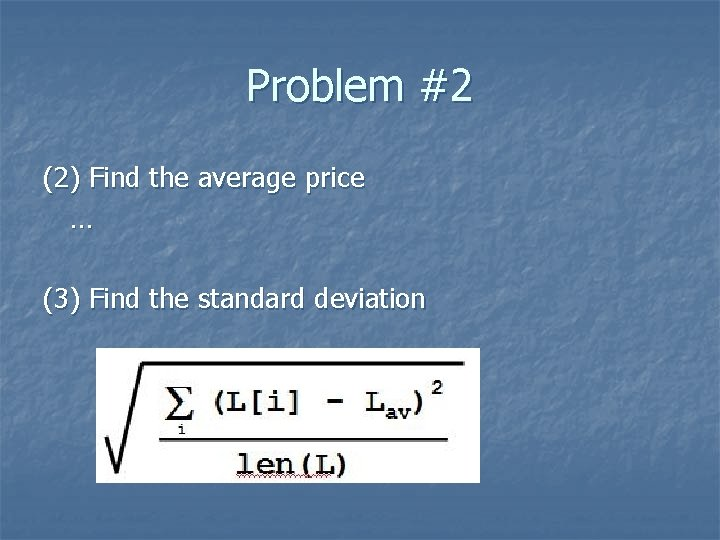Problem #2 (2) Find the average price … (3) Find the standard deviation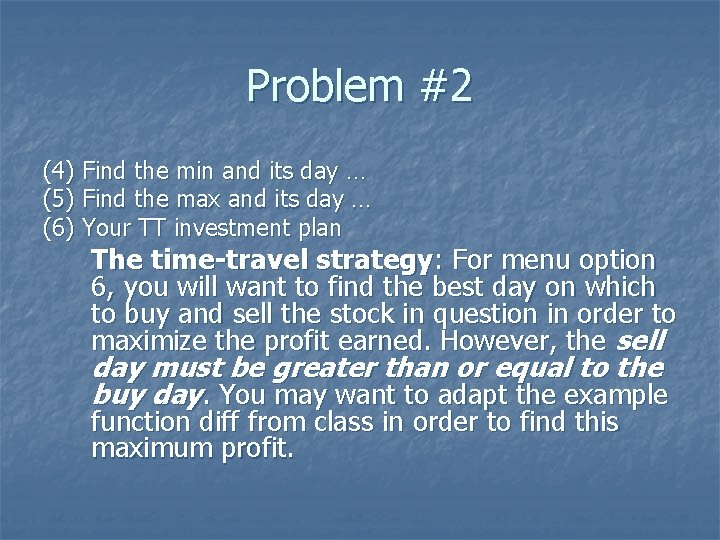Problem #2 (4) Find the min and its day … (5) Find the max and its day … (6) Your TT investment plan The time-travel strategy: For menu option 6, you will want to find the best day on which to buy and sell the stock in question in order to maximize the profit earned. However, the sell day must be greater than or equal to the buy day. You may want to adapt the example function diff from class in order to find this maximum profit.Problem #2 Other menu options… n print a warning message if the integer is not a valid menu option n quit if the user inputs #ask for input #get input While… #menu options #ask for input #get input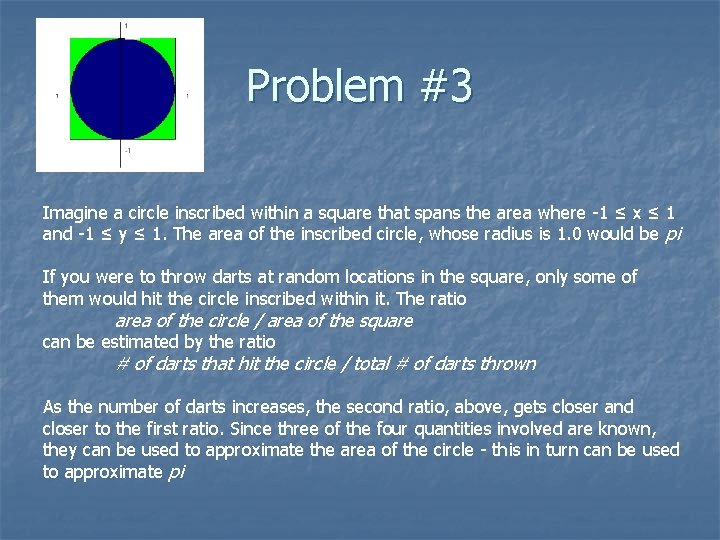Problem #3 Imagine a circle inscribed within a square that spans the area where -1 ≤ x ≤ 1 and -1 ≤ y ≤ 1. The area of the inscribed circle, whose radius is 1. 0 would be pi If you were to throw darts at random locations in the square, only some of them would hit the circle inscribed within it. The ratio area of the circle / area of the square can be estimated by the ratio # of darts that hit the circle / total # of darts thrown As the number of darts increases, the second ratio, above, gets closer and closer to the first ratio. Since three of the four quantities involved are known, they can be used to approximate the area of the circle - this in turn can be used to approximate piProblem #3 The for. Pi( n ) function takes in a positive integer n and should "throw" n darts at the square. Each time a dart is thrown, the function should print n n the number of darts thrown so far that have hit the circle the resulting estimate of pi def for. Pi(n) #initiate variables for i in …. : #use random to find an x and a y between -1 & 1 #make equation to find if the (x, y) coord is in the circle #if its in the circle, you’ve got a hit! #print the num of throws, num. of hits, estimate of piProblem #3 n n The while. Pi( error ) function, on the other hand, will take in a positive floating-point value, error, and should then continue to throw darts at the dartboard (the square) until the resulting estimate of pi is less than error. As with the for. Pi function, this function should print the number of darts thrown so far that have hit the circle the resulting estimate of pi def while. Pi(error): #initiate variables while (difference between your pi estimate and pi is greater than error ): #find x and y between -1 and 1 #calculate if x & y are in circle # if in circle you’ve got a hit! #print the num of throws, num. of hits, estimate of pi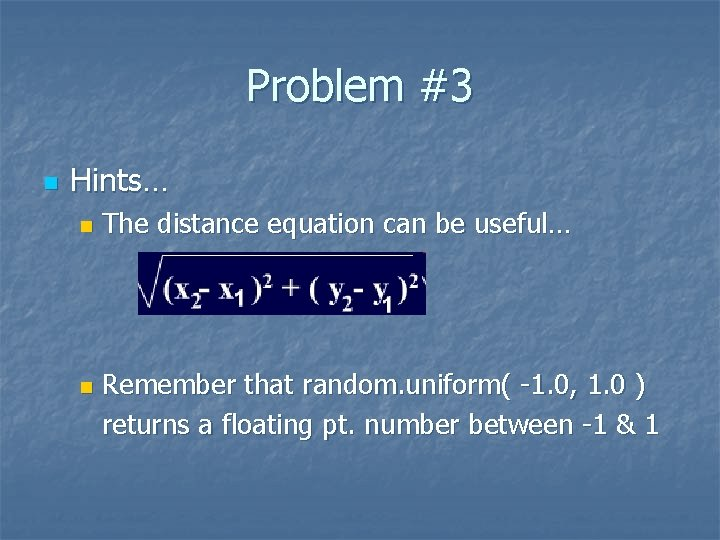Problem #3 n Hints… n n The distance equation can be useful… Remember that random. uniform( -1. 0, 1. 0 ) returns a floating pt. number between -1 & 1Extra Credit: Problem #4 Part a n This problem investigates the "Read-it-andweep" sequence: The sequence starts like this: 1, 11, 21, 1211, 111221, 312211, 13112221, . . . This sequence is called the Look and Say sequence, or sometimes, the "Read it and Weep" sequence. (Read it out loud and you will understand why. )Problem #4 Part a Write a helper function read. One( s ) that takes in a string of digits s and returns the string that represents the "reading" of s. For example: >>> read. One( '1' ) '11' >>> read. One( '11' ) '21' >>> read. One( '21' ) '1211' >>> read. One( '1211' ) '111221' n A special case to consider for read. One is the case when the input, s, has length 1. n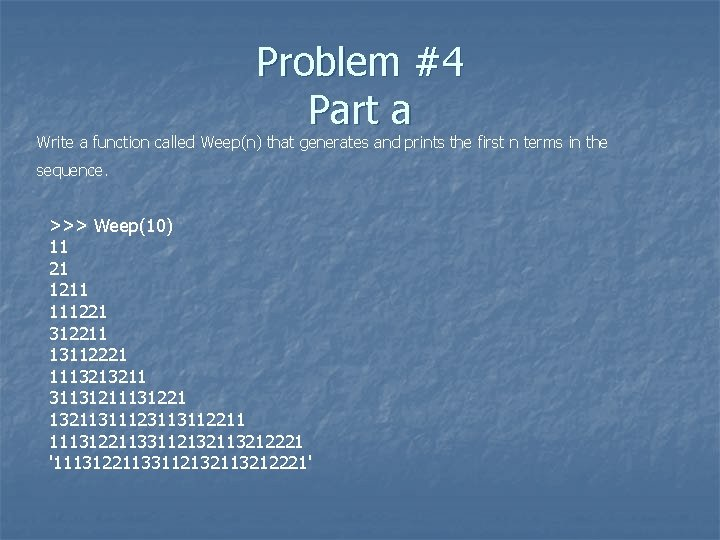Problem #4 Part a Write a function called Weep(n) that generates and prints the first n terms in the sequence. >>> Weep(10) 11 21 1211 111221 312211 13112221 1113213211 31131211131221 13211311123113112211 1113122113311213212221 '1113122113311213212221'Problem 4 Part b n The harmonic series is defined as: n n 1/1 + 1/2 + 1/3 + 1/4 +. . . This series can be shown to diverge; that is, the series goes to infinity. However, this series diverges very slowly. Write a function called n harmonic. N( num. To. Reach ) that returns the least number of terms that must be summed in order to reach or exceed num. To. Reach.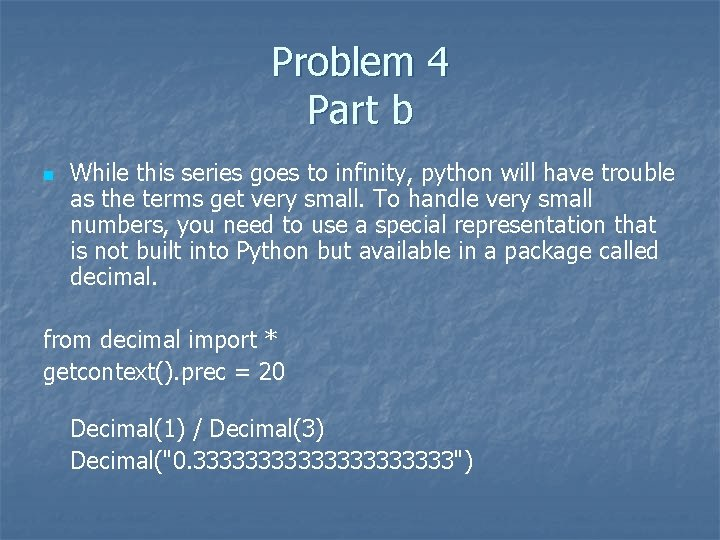Problem 4 Part b n While this series goes to infinity, python will have trouble as the terms get very small. To handle very small numbers, you need to use a special representation that is not built into Python but available in a package called decimal. from decimal import * getcontext(). prec = 20 Decimal(1) / Decimal(3) Decimal("0. 3333333333")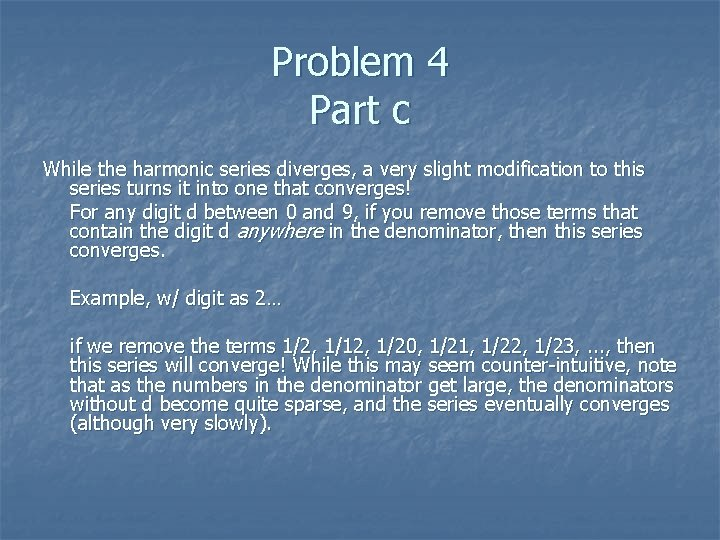Problem 4 Part c While the harmonic series diverges, a very slight modification to this series turns it into one that converges! For any digit d between 0 and 9, if you remove those terms that contain the digit d anywhere in the denominator, then this series converges. Example, w/ digit as 2… if we remove the terms 1/2, 1/12, 1/20, 1/21, 1/22, 1/23, . . . , then this series will converge! While this may seem counter-intuitive, note that as the numbers in the denominator get large, the denominators without d become quite sparse, and the series eventually converges (although very slowly).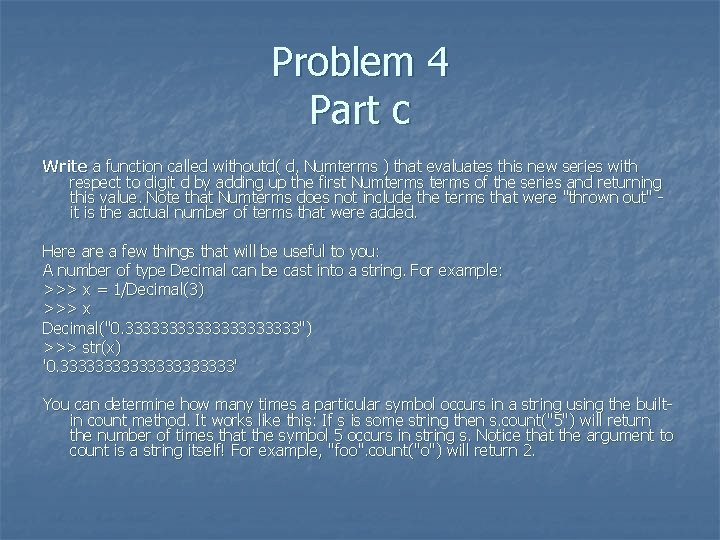Problem 4 Part c Write a function called withoutd( d, Numterms ) that evaluates this new series with respect to digit d by adding up the first Numterms of the series and returning this value. Note that Numterms does not include the terms that were "thrown out" - it is the actual number of terms that were added. Here a few things that will be useful to you: A number of type Decimal can be cast into a string. For example: >>> x = 1/Decimal(3) >>> x Decimal("0. 3333333333") >>> str(x) '0. 3333333333' You can determine how many times a particular symbol occurs in a string using the builtin count method. It works like this: If s is some string then s. count("5") will return the number of times that the symbol 5 occurs in string s. Notice that the argument to count is a string itself! For example, "foo". count("o") will return 2.